Lightwave systems discussed so far are based on a simple digital modulation scheme in which an electrical binary bit stream modulates the intensity of an optical carrier inside an optical transmitter (on-off keying or OOK). The resulting optical signal, after its transmission through the fiber link, falls directly on an optical receiver that converts it to the original digital signal in the electrical domain. Such a scheme is referred to as intensity modulation with direct detection (IM/DD). Many alternative schemes, well known in the context of radio and microwave communication systems, transmit information by modulating both the amplitude and the phase of a carrier wave. Although the use of such modulation formats for optical systems was considered in the 1980s, it was only after year 2000 that phase modulation of optical carriers attracted renewed attention, motivated mainly by its potential for improving the spectral efficiency of WDM systems. Depending on the receiver design, such systems can be classified into two categories. In coherent lightwave systems, transmitted signal is detected using homodyne or heterodyne detection requiring a local oscillator. In the so-called self-coherent systems, the received signal is first processed optically to transfer phase information into intensity modulations and then sent to a direct-detection receiver.

The motivation behind this phase encoding is two-fold. First, the sensitivity of optical receivers can be improved with a suitable design compared with that of direct detection. Second, phase-based modulation techniques allow a more efficient use of fiber bandwidth by increasing the spectral efficiency of WDM systems.

Both the amplitude and the phase of an optical carrier can be employed for encoding the information that need to be transmitted. In the case of IM/DD systems, a binary amplitude shift keying (ASK) format is employed such that the peak amplitude (or intensity) of the carrier takes two values, one of them being close to zero (also called the OOK format). In this section, we focus on phase-based modulation formats employed in modern lightwave systems.

#### 1. Encoding of Optical Signals

The electric field E(t) associated with an optical carrier has the form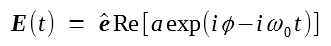where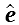is the polarization unit vector, a is the amplitude, φ is the phase, and ω0 is the carrier frequency. Introducing the complex phasor as A = ae, one can construct a constellation diagram in which the real and imaginary parts of A are plotted along the x and y axes, respectively. In the case of the OOK format, such a diagram has two points along the real axis, indicating that only the amplitude a changes from 0 to a1 whenever a bit 1 is transmitted (with no change in the phase).

The simplest PSK format is the one in which phase of the optical carrier takes two distinct values (see the figure below), typically chosen to be 0 and π. Such a format is called binary PSK, or BPSK. Coherent detection is a necessity for such a format because all information would be lost if the optical signal were detected directly without first mixing it coherently with a local oscillator. The use of PSK format requires the phase of the optical carrier to remain stable over a duration much longer than the bit duration, Tb = 1/B, at a given bit rate B. This requirement puts a stringent condition on tolerable spectral linewidths of both the laser and the local oscillator, especially when the bit rate is relatively small.The phase-stability requirement can be relaxed considerably by using a variant of the PSK format, known as differential PSK or DPSK. In the case of DPSK, information is coded in the phase difference between two neighboring bits. If only two phase values are used (differential BPSK or DBPSK), the phase difference Δφ = φk - φk-1 is changed by π or 0, depending on whether kth bit is a 1 or 0 bit. The advantage of a DPSK format is that the received signal can be demodulated successfully as long as the carrier phase remains relatively stable over a duration of two bits.

The BPSK format does not improve spectral efficiency because it employs only two distinct values of the carrier phase. If the carrier phase is allowed to take four distinct values, typically chosen to be 0, π/2, π, and 3π/2, one can transmit 2 bits simultaneously. Such a format is called quadrature PSK (QPSK), and its differential version is termed DQPSK. Figure (a) below, where the QPSK format is shown using a constellation diagram, can help in understanding how two bits can be transmitted simultaneously. As shown there, one can assign the four possible combinations of two bits, namely 00, 01, 10, 11, to four values of the carrier phase in a unique manner. As a result, the bit rate is effectively halved with the use of the QPSK (or DQPSK) format.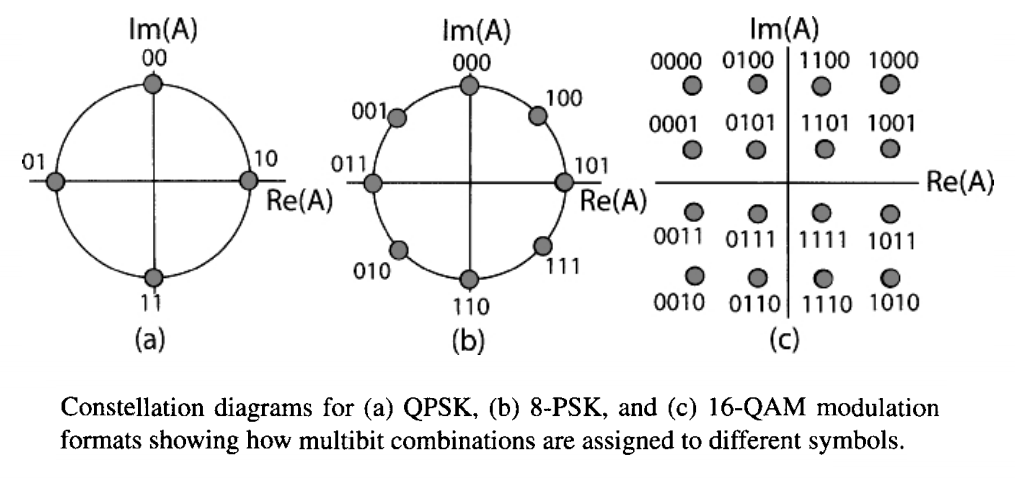The effective bit rate is called the symbol rate and is expressed in units of baud. In this terminology, well known in the areas of radio and microwave communications, phase values represent "symbols" that are transmitted, and their number M represents the size of the alphabet. The symbol rate Bs is related to the bit rate B by the simple relation B = log2(M)Bs. Thus, if the QPSK format with M = 4 is employed at Bs = 40 Gbaud, information will be transmitted at a bit rate of 80 Gb/s, resulting in a two-fold improvement in the spectral efficiency of a WDM system. Of course, the bit rate is tripled if one employs 8 distinct values of carrier phase using the so-called 8-PSK format. Figure (b) above shows the assignment of 3 bits to each symbol in this case.

Much more sophisticated modulation formats can be designed if the amplitude of the signal is also allowed to change from one symbol to next. An example is shown in the first figure (d) above, where the amplitude can take two possible values with four possible phases for each amplitude. Another example is shown figure (c) above, where 16 symbols lying on a square grid are employed to transmit 4 bits simultaneously. This modulation format is known 16-QAM, where QAM stands for quadrature amplitude modulation. Clearly, this approach can be extended to reduce the symbol rate even further at a given bit rate by extending the number M of symbols employed. It should be emphasized that the assignment of bit combinations to various symbols in the figure above is not arbitrary. A coding scheme, known as Gray coding, maps different bit combinations to different symbols in such a way that only a single bit changes between two adjacent symbols separated by the shortest distance in the constellation diagram. If Gray coding is not done, a single symbol error can produce errors in multiple bits, resulting in an increase in the system BER.

Spectral efficiency can be enhanced further by a factor of two by exploiting the state of polarization (SOP) of the optical carrier. In the case of polarization-division multiplexing (PDM), each wavelength is used to transmit two orthogonally polarized bit streams at half the original bit rate. It may appear surprising that such a scheme can work because the SOP of a channel does not remain fixed inside an optical fiber and can vary in a random fashion because of birefringence fluctuations. However, it is easy to see that PDM can be employed successfully as long as the two PDM channels at each wavelength remain close to orthogonally polarized over the entire link length. This can occur only if the effects of PMD and nonlinear depolarization remain relatively small over the entire link length. If coherent detection is employed at the receiver, it is possible to separate the two PDM channels with a suitable polarization-diversity scheme. The combination of QPSK (or DQPSK) and PDM reduces the symbol rate to one quarter of the actual bit rate and thus enhances the spectral efficiency by a factor of 4. Such a dual-polarization QPSK format is attractive because a 100-Gb/s signal can be transmitted over fiber links designed to carry 10-Gb/s signal with 50-GHz channel spacing and was in use for commercial systems by 2010.

One more design issue needs to be addressed. In the case of a purely phase-encoded signal, such as the QPSK format shown in figure (a) above, the amplitude or power of the data stream is initially constant with time when the NRZ format is employed because each symbol occupies the entire time slot allotted to it. This situation has two implications. First, the average power launched into each channel is enhanced considerably, an undesirable feature in general. Second, various dispersive and nonlinear effects induce time-dependent power variations during transmission of the data stream through the fiber that affect the system performance. An alternative is to employ a modulation format in which all symbol slots contain an optical pulse whose phase varies according to the data being transmitted. This situation is indicated by prefixing RZ to the format employed for data transmission (e.g., RZ-DQPSK).

#### 2. Amplitude and Phase Modulators

The implementation of any PSK format requires an external modulator capable of changing the optical phase in response to an applied voltage through a physical mechanism known as electrorefraction. Any electro-optic crystal with proper orientation can be used for phase modulation. A LiNbO3 waveguide is commonly used in practice. The phase shift δφ occurring inside the waveguide is related to the index change δn by the simple relation

δφ = (2π/λ)(δn)lm

where λ is the wavelength of the input light and lm is the modulator length. The index change δn is proportional to the applied voltage. Thus, any phase shift can be imposed on the optical carrier by applying the required voltage.

An amplitude modulator is also needed in most practical cases. It can be used to convert a CW signal from a DFB laser into a RZ pulse train. It can also be used to modulate the amplitude and phase of incident light simultaneously. A common design employs a Mach-Zehnder (MZ) interferometer for converting a voltage-induced phase shift into amplitude modulation of the input signal.

The following figure shows schematically the design of a LiNbO3 MZ modulator.The input field Ai is split into two equal parts at a Y junction, which are recombined back by another Y junction after different phase shifts have been imposed on them by applying voltages across two waveguides that form the two arms of a MZ interferometer. It is common to express these phase shifts in the form φj(t) = πVj(t)/Vπ, where Vj is the voltage applied across the jth arm (j = 1, 2) and Vπ is the voltage required to produce a π phase shift. This parameter is known for any LiNbO3 modulator and is typically in the range of 3 to 5 V. In terms of the two phase shifts, the transmitted field is given by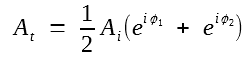The transfer function of the modulator is easily obtained in the formIt shows that a MZ modulator affects both the amplitude and the phase of the light incident on it. It can act as a pure amplitude modulator if we choose voltages in the two arms such that V2(t) = - V1(t) + Vb, where Vb is a constant bias voltage, because φ1 + φ2 then reduces to a constant. The power transfer function of the modulator in this case takes the form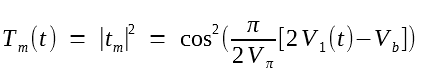Such a MZ modulator can act as a pure phase modulator that changes only the phase of input signal by an amount φ1(t) if the same voltage is applied to the two arms such that φ1 = φ2.

Although a single MZ modulator can change both the amplitude and phase of input light simultaneously by choosing the arm voltages V1 and V2 appropriately, it does not modulate the two quadratures independently. A solution is provided by a quadrature modulator realized by nesting three MZ modulators in a way shown in the figure below such that each arm of the outer MZ interferometer contains its own MZ modulator. By choosing the applied voltages appropriately, one can cover the entire complex plane in the constellation diagram.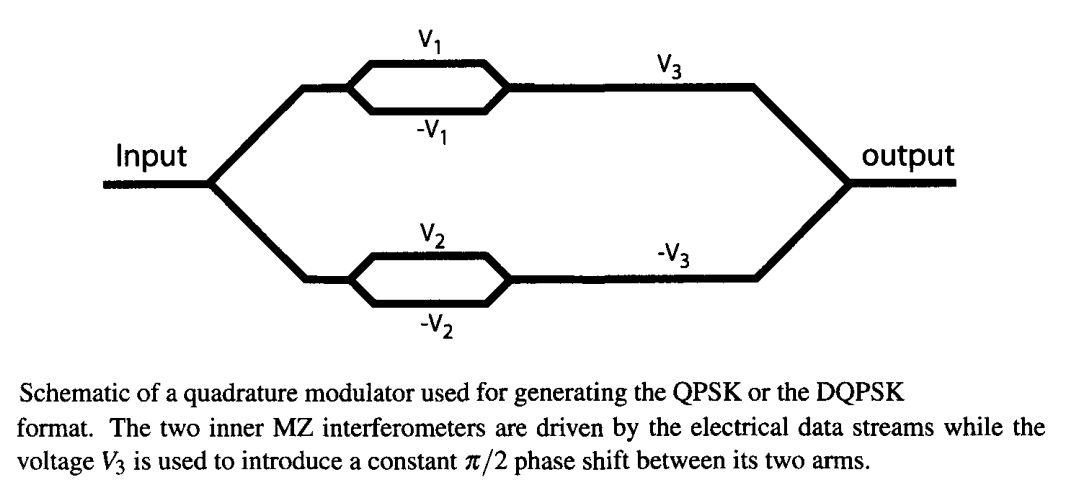As an example, consider the QPSK modulation format. In this case, two internal MZ modulators are operated in the so-called push-pull regime in which φ2 = - φ1. Further, the voltage is changed such that the transfer function tm takes values ±1, corresponding to two phase shifts of 0 and π, depending on the data bits being transmitted. The outer modulator is biased such that it produces a constant π/2 phase shift between the signals in its two arms. The output then has four possible phase shifts given by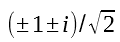which corresponds to four phase values of π/4, 3π/4, 5π/4, and 7π/4 and are suitable for creating a data stream with the QPSK modulation. A DQPSK symbol stream can also be created with such a modulator when the two internal MZ modulators are driven by an electrical signal that has been differentially encoded.